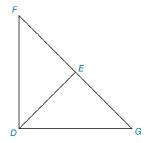Chapter 3.2, Problem 28E### Elementary Geometry for College St...

6th Edition
Daniel C. Alexander + 1 other
ISBN: 9781285195698

#### Solutions

Chapter
Section### Elementary Geometry for College St...

6th Edition
Daniel C. Alexander + 1 other
ISBN: 9781285195698
Textbook Problem
1 views

# In Exercise 27 to 29, prove the indicated relationship.Given: D E → bisects ∠ F D G ∠ F ≅ ∠ G Prove: E is midpoint of F G ¯

To determine

To prove:

The given statement.

Explanation

Given:

The following figure shows the given diagram.

Given that,

DE bisects FDG

FG

Approach:

By definition of ASA, if two angles and their included side of one triangle is congruent to two angles and their included side of second triangle, then triangle will be congruent.

The completed proof is shown in the following table.

 PROOF Statements Reasons 1. DE→ bisects ∠FDG 1. Given 2. ∠FDE≅∠GDE 2. If a ray divides an angle in to two ≅.∠s, then the ray bisects the right angle 3. ∠F≅∠G 3. Given 4. DG¯≅FD¯ 4. Transitive property of triangle 5. ΔFDE≅ΔEDG 5. ASA 6. FE¯≅EG¯ 6. CPCTC 7. E is midpoint of FG¯ 7

### Still sussing out bartleby?

Check out a sample textbook solution.

See a sample solution

#### The Solution to Your Study Problems

Bartleby provides explanations to thousands of textbook problems written by our experts, many with advanced degrees!

Get Started

#### In problems 45-62, perform the indicated operations and simplify. 52.

Mathematical Applications for the Management, Life, and Social Sciences

#### In Exercises 516, evaluate the given quantity. log1,000

Finite Mathematics and Applied Calculus (MindTap Course List)

#### f(x)=2xx+1; limxf(x); and limxf(x) x 1 10 100 1000 f(x) x 5 10 100 1000 f(x)

Applied Calculus for the Managerial, Life, and Social Sciences: A Brief Approach

#### Find f(x) if f(x)=1x2 and f(2) = 0. a) 1x+12 b) 1x12 c) 3x3+38 d) 3x338

Study Guide for Stewart's Single Variable Calculus: Early Transcendentals, 8th

#### The area of the shaded region is given by:

Study Guide for Stewart's Multivariable Calculus, 8th